# Using REPLACE and SUBSTITUTE functions in Excel - formula examples

The tutorial explains the Excel REPLACE and SUBSTITUTE functions with examples of uses. See how to use the REPLACE function with text strings, numbers and dates, and how to nest several REPLACE or SUBSTITUTE functions within one formula.

Last week we discussed various ways of using FIND and SEARCH functions within your Excel worksheets. Today, we will be taking a deeper look at two other functions to replace text in a cell based on its location or substitute one text string with another based on content. As you may have guessed, I am talking about the Excel REPLACE and SUBSTITUTE functions.

## Excel REPLACE function

The REPLACE function in Excel allows you to swap one or several characters in a text string with another character or a set of characters.

REPLACE(old_text, start_num, num_chars, new_text)

As you see, the Excel REPLACE function has 4 arguments, all of which are required.

• Old_text - the original text (or a reference to a cell with the original text) in which you want to replace some characters.
• Start_num - the position of the first character within old_text that you want to replace.
• Num_chars - the number of characters you want to replace.
• New_text - the replacement text.

For example, to change the word "sun" to "son", you can use the following formula:

`=REPLACE("sun", 2, 1, "o")`

And if you put the original word in some cell, say A2, you can supply the corresponding cell reference in the old_text argument:

`=REPLACE(A2, 2, 1, "o")`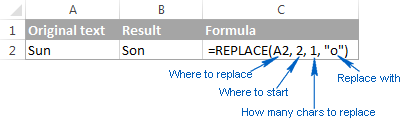Note. If the start_num or num_chars argument is negative or non-numeric, an Excel Replace formula returns the #VALUE! error.

### Using Excel REPLACE function with numeric values

The REPLACE function in Excel is designed to work with text strings. Of course, you can use it to replace numeric characters that are part of a text string, for example:

`=REPLACE(A2, 7, 4, "2016")`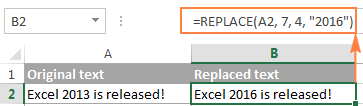Notice that we enclose "2016" in double quotes as you usually do with text values.

In a similar manner, you can replace one or more digits within a number. For example:

`=REPLACE(A4, 4, 4,"6")`

And again, you have to enclose the replacement value in double quotes ("6").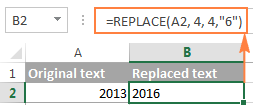Note. An Excel REPLACE formula always returns a text string, not number. In the screenshot above, notice the left alignment of the returned text value in B2, and compare it to the right-aligned original number in A2. And because it's a text value you won't be able to use it in other calculations unless you convert it back to number, for example by multiplying by 1 or by using any other method described in How to convert text to number.

### Using Excel REPLACE function with dates

As you have just seen, the REPLACE function works fine with numbers, except that it returns a text string :) Remembering that in the internal Excel system, dates are stored as numbers, you may try to use some Replace formulas on dates. Results would be quite embarrassing.

For instance, you have a date in A2, say 1-Oct-14, and you want to change "Oct" to "Nov". So, you write the formula REPLACE(A2, 4, 3, "Nov") that tells Excel to replace 3 chars in cells A2 beginning with the 4th char… and got the following result: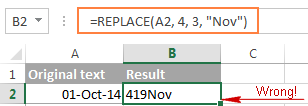Why's that? Because "01-Oct-14" is only a visual representation of the underlying serial number (41913) that represents the date. So, our Replace formula changes the last 3 digits in the above serial number to "Nov" and returns the text string "419Nov".

To get the Excel REPLACE function to correctly work with dates, you can convert dates to text strings first by using the TEXT function or any other technique demonstrated in How to convert date to text in Excel. Alternatively, you can embed the TEXT function directly in the old_text argument of the REPLACE function:

`=REPLACE(TEXT(A2, "dd-mmm-yy"), 4, 3, "Nov")`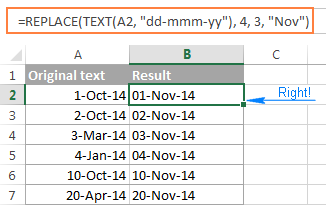Please remember that the result of the above formula is a text string, and therefore this solution works only if you are not planning to use the modified dates in further calculations. If you do need dates rather than text strings, use the DATEVALUE function to turn the values returned by the Excel REPLACE function back to dates:

`=DATEVALUE(REPLACE(TEXT(A2, "dd-mmm-yy"), 4, 3, "Nov"))`

### Nested REPLACE functions to do multiple replacements in a cell

Quite often, you may need to do more than one replacement in the same cell. Of course, you could do one replacement, output an intermediate result into an additional column, and then use the REPLACE function again. However, a better and more professional way is to use nested REPLACE functions that let you perform several replacements with a single formula. In this context, "nesting" means placing one function within another.

Consider the following example. Supposing you have a list of telephone numbers in column A formatted as "123456789" and you want to make them look more like phone numbers by adding hyphens. In other words, your goal is to turn "123456789" into "123-456-789".

Inserting the first hyphen is easy. You write a usual Excel Replace formula that replaces zero characters with a hyphen, i.e. adds a hyphen in the 4th position in a cell:

`=REPLACE(A2,4,0,"-")`

The result of the above Replace formula is as follows: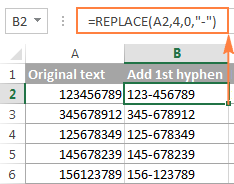Okay, and now we need to insert one more hyphen in the 8th position. To do this, you place the above formula within another Excel REPLACE function. More precisely, you embed it in the old_text argument of the other function, so that the second REPLACE function will handle the value returned by the first REPLACE, and not the value in cell A2:

`=REPLACE(REPLACE(A2,4,0,"-"),8,0,"-")`

As the result, you get the phone numbers in the desired formatting: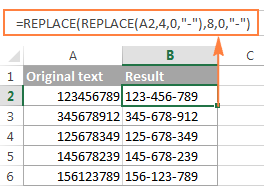In a similar manner, you can use nested REPLACE functions to make text strings look like dates by adding a forward slash (/) where appropriate:

`=(REPLACE(REPLACE(A2,3,0,"/"),6,0,"/"))`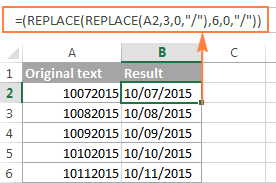Moreover, you can convert text strings into real dates by wrapping the above REPLACE formula with the DATEVALUE function:

`=DATEVALUE(REPLACE(REPLACE(A2,3,0,"/"),6,0,"/"))`

And naturally, you are not limited in the number of functions you can nest within one formula (the modern versions of Excel 2010, 2013 and 2016 allow up to 8192 characters and up to 64 nested functions in a formula).

For example, you can use 3 nested REPLACE functions to have a number in A2 appear like date and time:

`=REPLACE(REPLACE(REPLACE(REPLACE(A2,3,0,"/") ,6,0,"/"), 9,0, " "), 12,0, ":")`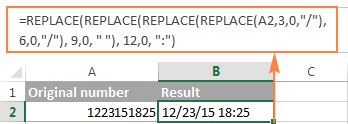### Replacing a string that appears in a different position in each cell

So far, in all the examples we have been dealing with values of a similar nature and have made replacements in the same position in each cell. But real-life tasks are often more complicated than that. In your worksheets, the characters to be replaced may not necessarily appear in the same place in each cell, and therefore you will have to find the position of the first character that should be replaced. The following example will demonstrate what I'm talking about.

Supposing you have a list of email addressing in column A. And the name of one company has changed from "ABC" to, say, "BCA". So, you have to update all of the clients' email addressing accordingly.

But the problem is that the client names are of different length, and that is why you cannot specify exactly where the company name begins. In other words, you do not know what value to supply in the start_num argument of the Excel REPLACE function. To find it out, use the Excel FIND function to determine the position of the first char in the string "@abc":

`=FIND("@abc",A2)`

And then, supply the above FIND function in the start_num argument of your REPLACE formula:

`=REPLACE(A2, FIND("@abc",A2), 4, "@bca")`

Tip. We include "@" in our Excel Find and Replace formula to avoid accidental replacements in the name part of email addresses. Of course, there's a very slim chance that such matches will occur, and still you may want to be on the safe side.

As you see in the following screenshot, the formula has no problem with finding and replacing the old text with the new one. However, if the text string to be replaced is not found, the formula returns the #VALUE! error: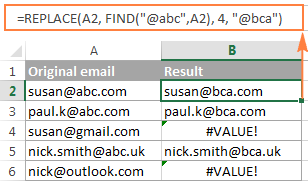And we want the formula to return the original email address instead of the error. So, let's enclose our FIND & REPLACE formula in the IFERROR function:

`=IFERROR(REPLACE(A2, FIND("@abc",A2), 4, "@bca"),A2)`

And this improved formula works perfectly, doesn't it?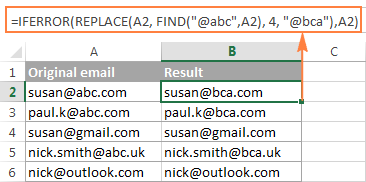Another practical application of the REPLACE function is to capitalize the first letter in a cell. Whenever you deal with a list of names, products, and the like, you can use the above-linked formula to change the first letter to UPPERCASE.

Tip. If you want to make the replacements in the original data, an easier way would be using the Excel FIND and REPLACE dialog.

## Excel SUBSTITUTE function

The SUBSTITUTE function in Excel replaces one or more instances of a given character or text string with a specified character(s).

The syntax of the Excel SUBSTITUTE function is as follows:

SUBSTITUTE(text, old_text, new_text, [instance_num])

The first three arguments are required and the last one is optional.

• Text - the original text in which you want to substitute characters. Can be supplied as a test string, cell reference, or a result of another formula.
• Old_text - the character(s) you want to replace.
• New_text - the new character(s) to replace old_text with.
• Instance_num - the occurrence of old_text you want to replace. If omitted, every occurrence of the old text will be changed to the new text.

For example, all of the below formulas substitute "1" with "2" in cell A2, but return different results depending on which number you supply in the last argument:

`=SUBSTITUTE(A2, "1", "2", 1)` - Substitutes the first occurrence of "1" with "2".

`=SUBSTITUTE(A2, "1", "2", 2)` - Substitutes the second occurrence of "1" with "2".

`=SUBSTITUTE(A2, "1", "2")` - Substitutes all occurrences of "1" with "2".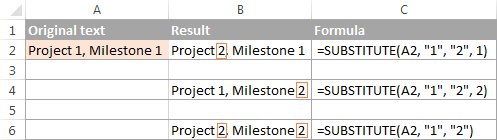In practice, the SUBSTITUTE function is also used for removing unwanted characters from cells. For real-life examples, please see:

Note. The SUBSTITUTE function in Excel is case-sensitive. For example, the following formula replaces all instances of the uppercase "X" with "Y" in cell A2, but it won't replace any instances of the lowercase "x".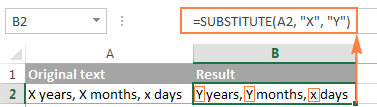### Substitute multiple values with a single formula (nested SUBSTITUTE)

As is the case with the Excel REPLACE function, you can nest several SUBSTITUTE functions within a single formula to do several substitutions at a time, i.e. substitute several characters or substrings with a single formula.

Supposing you have a text string like "PR1, ML1, T1" in cell A2, where "PR" stands for "Project, "ML" stands for "Milestone" and "T" means "Task". What you want is to replace the three codes with full names. To achieve this, you can write 3 different SUBSTITUTE formulas:

`=SUBSTITUTE(A2,"PR", "Project ")`

`=SUBSTITUTE(A2, "ML", "Milestone ")`

`=SUBSTITUTE(A2, "T", "Task ")`

And then nest them into each other:

`=SUBSTITUTE(SUBSTITUTE(SUBSTITUTE(A2,"PR","Project "),"ML","Milestone "),"T","Task ")`

Notice that we've added a space at the end of each new_text argument for better readability.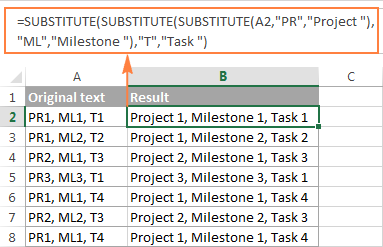To learn other ways to replace multiple values at a time, please see How to do mass find and replace in Excel.

## Excel REPLACE vs. Excel SUBSTITUTE

The Excel REPLACE and SUBSTITUTE functions are very similar to each other in that both are designed to swap text strings. The differences between the two functions are as follows:

• SUBSTITUTE replaces one or more instances of a given character or a text string. So, if you know the text to be replaced, use the Excel SUBSTITUTE function.
• REPLACE changes characters in a specified position of a text string. So, if you know the position of the character(s) to be replaced, use the Excel REPLACE function.
• The SUBSTITUTE function in Excel allows adding an optional parameter (instance_num) that specifies which occurrence of old_text should be changed to new_text.

This is how you use the SUBSTITUTE and REPLACE functions in Excel. Hopefully, these examples will prove useful in solving your tasks. I thank you for reading and hope to see on our blog next week!

REPLACE and SUBSTITUTE formula examples (.xlsx file)

## You may also be interested in

1. how can I change this format to 04-05-2021 AM 2:45:55 to 04-05-2021 2:45:55 AM

• Hello!
If your values are written as date, then apply a custom date format as described in this article.
If your date is written as text, use the formula

=REPLACE(LEFT(A1,LEN(A1)-8),SEARCH(" ",A1,1),1, RIGHT(A1,LEN(A1)-13)&" ")

2. The string is an NCAA basketball tournament game schedule as follows:

6:30 pm — No. 16 N.C. Central vs. No. 16 Texas Southern (West/Dayton, OH), TruTV

Where each team name can be one, two or three words as follows:

4:00 pm — No. 5 Ohio State vs. No. 12 South Dakota State (West/Boise, ID), TNT

Have used substitute to replace all unnecessary characters so that desired data elements are all separated by a single space...

6:30 16 N.C. Central 16 Texas Southern West Dayton OH TruTV

4:00 5 Ohio State 12 South Dakota State West Boise ID TNT

Each space separated element (other than team name) needs to become its own field in the record. Team name is a separate field. If the pm time could be appropriately valued numerically, that would be a bonus for sure.

Thank you for any suggestions.

Yours is an excellent site. I have no problem purchasing a license for your tool set, but I'm not sure if the mechanics are there to execute this very specific string extract problem...

• Hi Sam,

You can use our Split Text tool to split your strings by this mask:
* — No. * * vs. No. * * (*), *

The following image shows the settings and outcomes:

Also, you can download a trial version using this link and test it on real data. Perhaps, you will need to additionally remove some unwanted characters by using the Remove tool.

If you have any further questions or concerns, just let me know :)

3. Hi,

its possible to rename needed phraze from Cell1 to needed phraze from Cell2?

for example -->
=SUBSTITUTE(A1;"cell1 phraze";("phraze from different cell2"))

is there option to read information from other cell?

• Hi,
If "Cell1" is cell A1, then this is not possible. If A1 says "cell1 phraze" then where do you want to write the formula that will change this phrase?
If this is not what you wanted, please describe the problem in more detail.

4. I need to copy a delimited cell into another cell, but only the values between the delimiters that are less than 30 characters long.

E.g.

Reference cell: blue||green||Adding another tag||this tag is toooooooooooooooooooooooo long||this tag is good||short tag

I want to extract all of the tags that are under 30 characters and put them in the next cell so it would be:
blue||green||Adding another tag||this tag is good||short tag

I know how to do this from a column, but need to figure out how to do it from a delimited list and am having no luck.

• Hello!
You need to split the text into cells and then select the ones you want. I recommend paying attention to the tool Split Text. It is available as a part of our Ultimate Suite for Excel that you can install in a trial mode and check how it works for free. If something is still unclear, please feel free to ask.

5. =SUBSTITUTE(A2,"PR", "Project ")

Instead of substituting PR with “Project” how would you substitute PR with the content of a cell ?

Example content of A9 = “Happy”
Result= PR becomes Happy

Need to do this with a list of names that will have their own unique URL

• Hello!

=SUBSTITUTE(A2,"PR", A9)

If this is not what you wanted, please describe the problem in more detail.

6. How can I substitute max to AJanMAX

7. How can i convert " 19:00-20:00-UAE " to " 1900 - 2000" format.

• Hello!
You can search for the desired word in 3 steps. To find an exact match for a word, add a space after the word in the search box. Then repeat your search and add a space before the word. After that, search for the word and use the "Match entire cell contents" option.

9. Hello,

I am looking for a formula that will convert seconds into weeks, days, hours, minutes and a way to only show these if the value is not 0. So for example, 13500seconds would convert to 3h 45m.

Thank you!

• Hello!
To convert seconds to time, divide by 86400.

10. Excel has empty commas in one column e.g. ,, OR ,,, OR ,,,, they are not same number but all are empty.

We want to add text to other columns e.g. "A" "B" "C" etc.

We want to have a substitute formula that will replace all spaces in column one with text available in other columns.

So if there are two ,, we want to replace with A,B,C
if there are three ,,, we want to replace with A, B, C, D
if there is one then replace with A,B

how can we do this in excel? Thanks in advance!

• Hello!

=LEFT("ABCDEFGHIJKLMNOPQRSTUVWXYZ",LEN(A1)+1)

But if you want to replace commas with letters in the first column, then this is only possible with a VBA macro.

11. Hi!

I have column of numbers formatted as follows:

412.65
11.987
6.960.264
199.246
0
90.97
1194.2
90.375
7.8
1.001.479

The first period (.) from the right is a decimal point (we use commas), the other points are thousand seperators. The fields with three digits next to the last period are seen by Excel as whole numbers, the others as text. For example 1.001.479 is seen as one million, one thousand seventy nine, and not as 1000,479. 412.65 is seen as text.

Is there a formule to convert this column into proper numbers, the result should be:

412,65
11,987
6960,264
199,246
0
90,97
1194,2
90,375
7,8
1001,479

Basically, the most right period is a comma, all other periods removed?

Erik

• Hello!

=SUBSTITUTE(IFERROR(SUBSTITUTE(A1,".",",",LEN(A1) - LEN(SUBSTITUTE(A1,".",""))),A1),".","")

Hope this is what you need.

• Thanks, Alexander, that does the trick!

12. I want to replace time format i.e. for example 09:10:32 with word P and if the cell is blank with A , kindly help me with the formula

• Hi,
An Excel formula can change the value only in the cell that it is pasted in. If you need to change the content on the cell that has some value in it, you’ll need to use a VBA macro.

13. I Want to do a find and replace in formula form. I get Dates sent to me in a specific column " dd/mm/yyyy hh:mm:ss AM/PM". when I past information in to my workbook I want to format that column for it to Automatically change to "ddd hh:mm:ss AM/PM

Any assistance would be appreciated.

• Hello!
Set your column to "ddd hh:mm:ss AM/PM" format. When you paste values there, use Paste Special - Values. You can use the key combination CTRL + ALT + V then V and ENTER again.

14. Hello Alex,
How do I change the first zeros in numbers without affecting zeros that are in other positions; for example in the following values: 0Z105, 0Z1025, 0X 11605, 0Y112008; how do I replace only the zeros that come before letters Z,Z,X and Y? Many thanks in advance.

15. How do I Substitute part of a column for what is in another column. For example, Column E5 says ""Client Name" Branding". I want to substitute the "client name" in E5 for what is in Column A4, which is Zion. So I want it to say "Zion Branding". Column A4 is a pivot table so that I can change the client name as desired and it would change E5 to read the selected client name and then branding.

16. how to change below text with quantity (Q:100Adam-USA) to (Adam-USA) & it's copy for one column...???

• Hello!
If I understand your task correctly, the following formula should work for you:

=SUBSTITUTE((CONCAT(IF(NOT(ISNUMBER(--MID(SUBSTITUTE(A3,"Q:","",1), ROW(\$1:\$93),1))), MID(SUBSTITUTE(A3,"Q:","",1), ROW(\$1:\$93),1),"")))," ","")

• =REPLACE(text ,2,5,"")

17. Thank you!

18. How do you substitute the character " ?? Excel returns with invalid, when you try to create formula =SUBSTITUTE(A4,""","") to remove all " in your text.

19. Hi,
The substitute formula is picking up part words instead of whole words. How do I over come this?

• Hello!
Unfortunately, without seeing your data it is difficult to give you any advice. Please provide me with an example of the source data and the expected result. Please specify what formula you used and what problem or error occurred.

20. I am trying to concatente & substitute within the same fomula. I have worked out each formula separately, but I cannot put them together into the one formala - can you help?
=SUBSTITUTE(H6," ","_")
=CONCATENATE(H7,".jpg")

I'd be very grateful for your help.
Kind regards
Leeanne

• Hello!
If I understand your task correctly, the following formula should work for you:

=CONCATENATE(SUBSTITUTE(H6," ","_"),".jpg")

Hope this is what you need.

21. Good day,

Is it possible to have the information in the first tabe replaced with new information on the next line in tab 2.

For example A3 is equal to 1991 and is extracted from tab (sheet 2) B4. When I add information in tab (sheet 2) B5 i want that to change A3.

• Hello!
You didn't say exactly how you want to change A3 when B5 changes. But the formula might be something like this:

=IF(Sheet2!B5="",Sheet2!B4,Sheet2!B4+Sheet2!B5)

22. How do I change the item codes of a given list of products. The item/ product descriptions remain unchanged but new item/ product descriptions have been assigned to all the products.

• Hello!
I’m sorry but your task is not entirely clear to me. For me to be able to help you better, please describe your task in more detail. Please specify what you were trying to find, what formula you used and what problem or error occurred. Give an example of the source data and the expected result.
It’ll help me understand it better and find a solution for you.

23. I have the letters iii that repeat in various cells throughout a workbook. I would like to replace iii with a series of number where I can assign what number goes in for the first iii found and each subsequent iii gets replaced with a "+1" number. For instance, the first iii would be replaced with 300, the second instance of iii would be replaced with 301, the third with 302 and so forth.

24. How do I replace a certain list with another list? Thanks

25. Hi,
I want change the commas and replace as semicolons only outside brackers. But i dont want to change the commas within brackets.

// Identity information (name, employee ID, date of birth, age, gender) , Home contact information , Businnes Contact (employee name, address) //

Thanks
Deepa

• Hello!
It is very difficult to solve your problem with a formula.
We have a tool that can solve your task in a couple of clicks: Ablebits Data - Split Text.
You can split the text into columns. Use brackets () as delimiters. You can then replace the comma with a semicolon in the columns you want. With the Merge Cells tool, you can merge text into one cell again.
This tool is available as a part of our Ultimate Suite for Excel that you can install in a trial mode and use for free: https://www.ablebits.com/files/get.php?addin=xl-suite&f=free-trial
If you have any (other) questions, don't hesitate to ask.

26. Net Price
4,650,000.00 INR
1,550,000.00 USD
2,460,000.00 ERUO

HOW TO SLOW THIS

• Hello!
I’m sorry but your task is not entirely clear to me. For me to be able to help you better, please describe your task in more detail. Please specify what you were trying to find, what formula you used and what problem or error occurred. Give an example of the source data and the expected result.
It’ll help me understand it better and find a solution for you. Thank you.

27. Hi, can some one help me please,
i have a excel that in column A contains 22 digit numbers data,example (123496789123456178912).
this number represents alot of staff ( location of sales type etc..)
if the 4th character is "4" this means DE_SALES if "5" means AT_SALES
if the 5th character is "9" this means PICKEDUP if "0" DELIVERY
what is the best formula to use

• Hello!
To extract any character or multiple characters from text, use the MID function. Read more here. Then you can use the IF function to check the condition.
I hope this will help

28. how to replace
\$23,282 into \$23,838

29. How to replace
search.auckland.ac/search?allPage=1 to search.auckland.ac.nz/search?allPage=1
search.auckland.ac/search?allPage=1 to search.auckland.ac.nz/search?allPage=2
search.auckland.ac/search?allPage=1 to search.auckland.ac.nz/search?allPage=3
search.auckland.ac/search?allPage=1 to search.auckland.ac.nz/search?allPage=4
till page=74

• Hello Selvam!
If the list is in column A and starts with A1, then in B1 use the formula

=LEFT(SUBSTITUTE(A1,".ac",".ac.nz"), SEARCH("=",SUBSTITUTE(A1,".ac",".ac.nz"),1))&ROW()

After that you can copy this formula down along the column.
Hope this is what you need.

30. Can I change a cell value in a table from another cell

• Hello Kalpesh!
The Excel function can’t change values in other cells, it returns a value only in a cell where it is written. You'd better use VBA to solve your task.

31. Hello,
Can we place our new text in other cell than the one with the formula?

32. I am trying to convert text into time. I am using the TIMEVALUE function and have gotten 1/2 way there so far. Problem is Excel doesn't recognize my data as time because when it was exported, there is not a space between the time and p or a (Ex: 10:08p and 6:31a). I entered the formula: =TIMEVALUE(SUBSTITUTE(H3,"p"," pm") and it worked. The problem I have is that I don't know how to enter the formula to also change the "a" to " am" when this occurs in the column. Hoping someone can offer me assistance. Thank you!

33. Hi,

Is it possible to use the substitute function in an array, where there are other symbols or words intercalated? For a translation, as below:
Cell A2 : I want to go home.
Cell B2 : Je veux aller à la maison.
Cell C3 : I [slot] want [slot] to go [slot] home. [slot]

Can I bring my translation somehow in D2 with the slots appearing, not necessarily at the appropriate place, but at least to be within the words?

Thank you so much for your help!
N.

34. Hello Svetlana,
Very Good and helpful Article on Replace & Substitute function.
My question is: Can we use an Array Set for;
SUBSTITUTE(text, old_text, new_text, [instance_num])
OLD TEXT: 0?1-GR&*88945.@
SUBSTITUTE("0?1-GR&*88945.@", {"?";"-";"&";"*";".";"@"}, {"";"";"";"";"";""})
NEW TEXT: 01GR88945
CAN WE DO Like this to Get Results without using Nested Substitute Function.
Thank you

35. Hi sir
i need Starting first character in a cell should alphabets or numeric if other Character like special character should replace any formula could Remove

36. how to replace 1, 2,4 to G+0, G+1,G+3 respectively

37. I have data coming in where A1 is a network name from A2 until a variable amount of lines the cells have an asterisk. Then a space another network name and then more asterisks for an variable amount of lines, then another network name and it continues for about 10K total lines in the sheet. I am trying to replace the asterisk with the network name proceeding its section without doing it manually. I've tried search/replace, failed at a macro, and a few other things.

38. thank u very much , your tutorial is very helpfull ...

39. I am trying to create a data matrix. I want to be able to replace any instance of the number 1 in a row with "=b2" So that any instance of 1 in row b will return whatever value I put in cell b2.

I would to be able to drag this formula down or across as the data set I am working with is roughly 600 cells by 300 cells.

I have been accomplishing the same task by highlighting cell b3 to the end of the data field and doing - FIND 1 and REPLACE with =b2. But I must do this line by line manually

Thanks

KAS

40. =SUBSTITUTE(text, old_text, new_text)

if: a=!, b=@, c=#,... x=>, y=?, z=~, " "=" "
then: abcdefghijklmnopqrstuvwxyz ... try this out
equals: !@#\$%^&*()-=+[]\{}|;:/?~ ... ;}? ;*(| ]:;

RULES:
------
(1) text to substitute is in cell A1
(2) max 64 substitution levels (the formula below only has 27 levels [alphabet + space])
(2) "old_text" cannot also be a "new_text" (ie: if a=z .: z cannot be "old text")
---so if a=z,b=y,...y=b,z=a, then the result is
---abcdefghijklmnopqrstuvwxyz = zyxwvutsrqponnopqrstuvwxyz (and z changes to a then changes back to z) ... (pattern starts to fail after m=n, n=m... and n becomes n)

The formula is:

=SUBSTITUTE(SUBSTITUTE(SUBSTITUTE(SUBSTITUTE(SUBSTITUTE(SUBSTITUTE(SUBSTITUTE(SUBSTITUTE(SUBSTITUTE(SUBSTITUTE(SUBSTITUTE(SUBSTITUTE(SUBSTITUTE(SUBSTITUTE(SUBSTITUTE(SUBSTITUTE(SUBSTITUTE(SUBSTITUTE(SUBSTITUTE(SUBSTITUTE(SUBSTITUTE(SUBSTITUTE(SUBSTITUTE(SUBSTITUTE(SUBSTITUTE(SUBSTITUTE(SUBSTITUTE(A1,"a","!"),"b","@"),"c","#"),"d","\$"),"e","%"),"f","^"),"g","&"),"h","*"),"i","("),"j",")"),"k","-"),"l","="),"m","+"),"n","["),"o","]"),"p","\"),"q","{"),"r","}"),"s","|"),"t",";"),"u",":"),"v","/"),"w",""),"y","?"),"z","~")," ","_")

41. ...i am trying to do a reverse score formula for a data...how do i replace numbers without having the formula repeat back on itself? so for example, if a participant answers a "1" to a question, i want the answer in the cell to come up as "5"...i want the numbers reversed for 1 through 5 (i.e. i want 1=5, 2=4, 3=3, 4=2, 5=1).
=substitute(substitute(substitute(substitute(substitute(B5,"1","5"),"2","4"),"3","3"),"4","2"),"5","1")
what i ended up creating was a loop, i assume...because each "1" ended up being a "1" (because it made the initial change to "5", and then further along the formula changed that "5" into a "1"), and each "2" became a "2" (for the same reason). 3 4 and 5 were correct (because those numbers didnt show up again in the first position of the number string inthe formula).
So, how do i write the formula so i dont have a loop for those first two numbers?
thanks!

• You can use formula
=Max(A:A)+1-A5

42. Excellent article explaining the REPLACE & SUBSTITUTE functions, and I was able to correct some phone numbers using the REPLACE example in the article. But what I need to do now, is replace the individual cells with the corrected formatting (i.e. phone number without formatting to phone number with appropriate formatting). The column that has the original values has some cells with correct data and other cells with the incorrectly formatted phone numbers. Is there a simple way (using a formula?) to replace the new formatted number (in column C) with the incorrectly formatted number (in column B). Not every number in the column is incorrect, only about 100 of a total of about 700 entries. I hope I was clear.

43. 169 #N/A
169 #N/A
169 #N/A
169 Punjab National Bank
169 #N/A
169 #N/A

i want to have punjab national bank in all the other places where it is #N/A. please share logic to write in other column

• ctrl+H
find #N/A
Replace Punjab Nation Bank

44. How do i replace cell with ___AHEJ7224 with ___EJ8899 for example, i just want to replace all text in a column with new text leaving the underscore's.

45. Sir,
thank you so much i found the ans. in my problem the last example is the ans.

46. Sir,
possible to substitute a two different word or text? example in cell no A5 i would like to write ether PASS or Fail, and for my substitute cell A8 is YES or NO.
to make it clear i want to write PASS in my old text A5, the result in my substitute cell A8 will be YES, but if i write in my A5 cell is FAIL the result must be in A8 cell is NO.
IS THIS POSSIBLE? thank you so much.

Regards,
JPD

• Hi Jophet,
You can use if Function to return value Yes if text A5 is Pass while NO if the cell A5 is Fail e.g =IF(A5="PASS","YES","NO").
Thank you.

47. How to replace 784.1991.1796026.2 to 784-1991-1796026-2

• =SUBSTITUTE(YourCell,".","-")

• U can Also Use Find and Replace key.
eg. ctrl+H
FIND . AND REPLACE -

48. Thanks a lot.....
I've found my solution.. It really helps me much

49. When I import names from a SharePoint list I get the name, a semicolon, and then a number (example: Smith, John;#1234;#Jones,Mary:#567). This number is probably assigned by SharePoint when you add someone so they range from single digits to 5 digits for our company. I am trying to eliminate the "#" and the numbers. I can replace the "#" with a space using the substitute function but am having problems replacing the numbers due to the fact they are not a fixed number of digits. Also some cells could be a single name while others could have five or six names. Any insights?

• =TEXTJOIN("",TRUE,IF(ISERR(MID(A1,ROW(INDIRECT("1:"&LEN(A1))),1)+0),MID(A1,ROW(INDIRECT("1:"&LEN(A1))),1),""))